# RD Sharma Solutions for Class 11 Chapter 23 - The Straight Lines Exercise 23.5

In this exercise, we shall deal with one of the different forms of the equation of a straight line i.e., two-point form of a line with suitable examples, for better understanding of the concepts. Numerous solved examples are present before the actual exercise to help students understand the method of solving problems in a shorter duration. By solving the textbook problems created by experts, students can boost their exam preparation. The solutions are explained in simple language to help students understand the concepts at ease. Students can access and download for free the RD Sharma Class 11 Maths Solutions pdf, from the links provided below.

## Download the pdf of RD Sharma Solutions for Class 11 Maths Exercise 23.5 Chapter 23 – The Straight Lines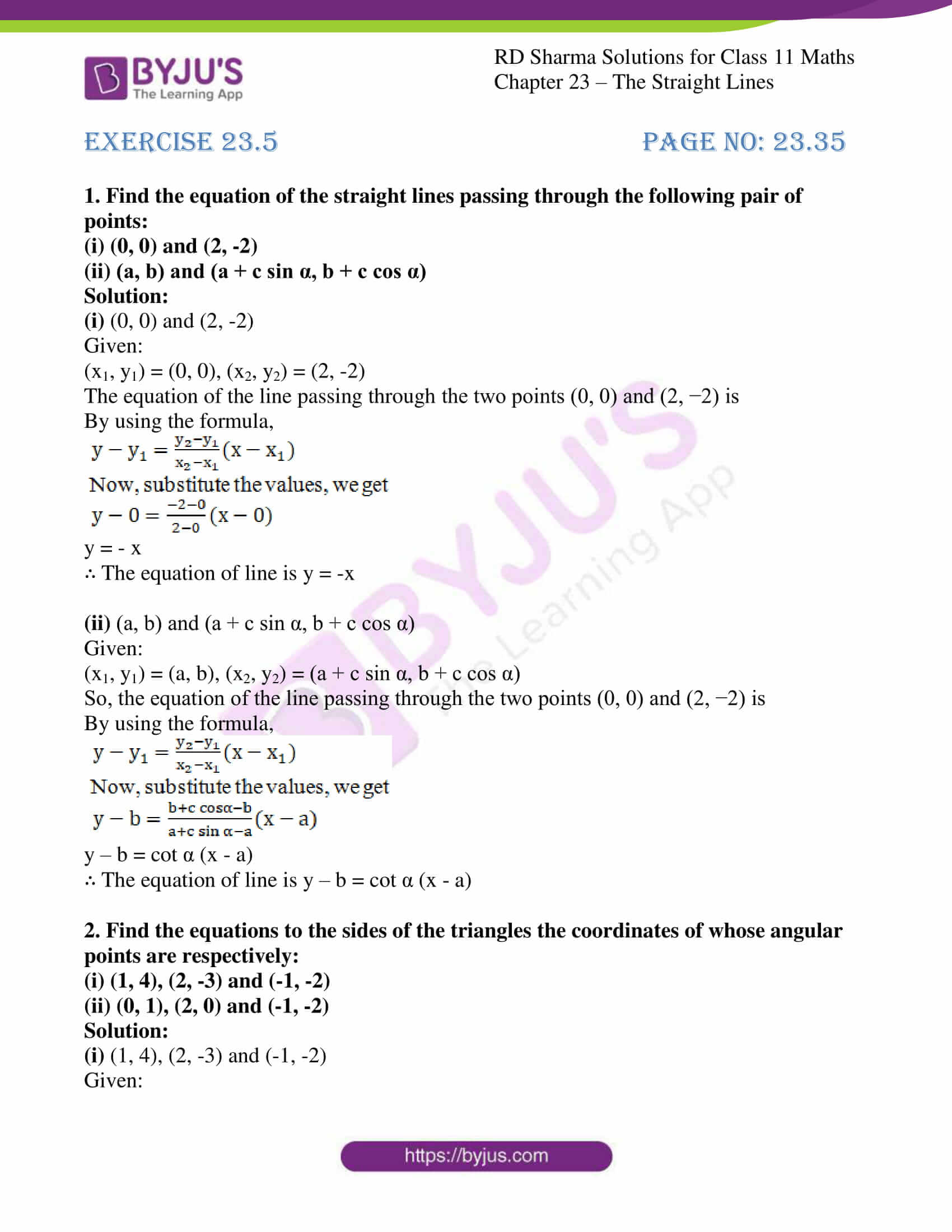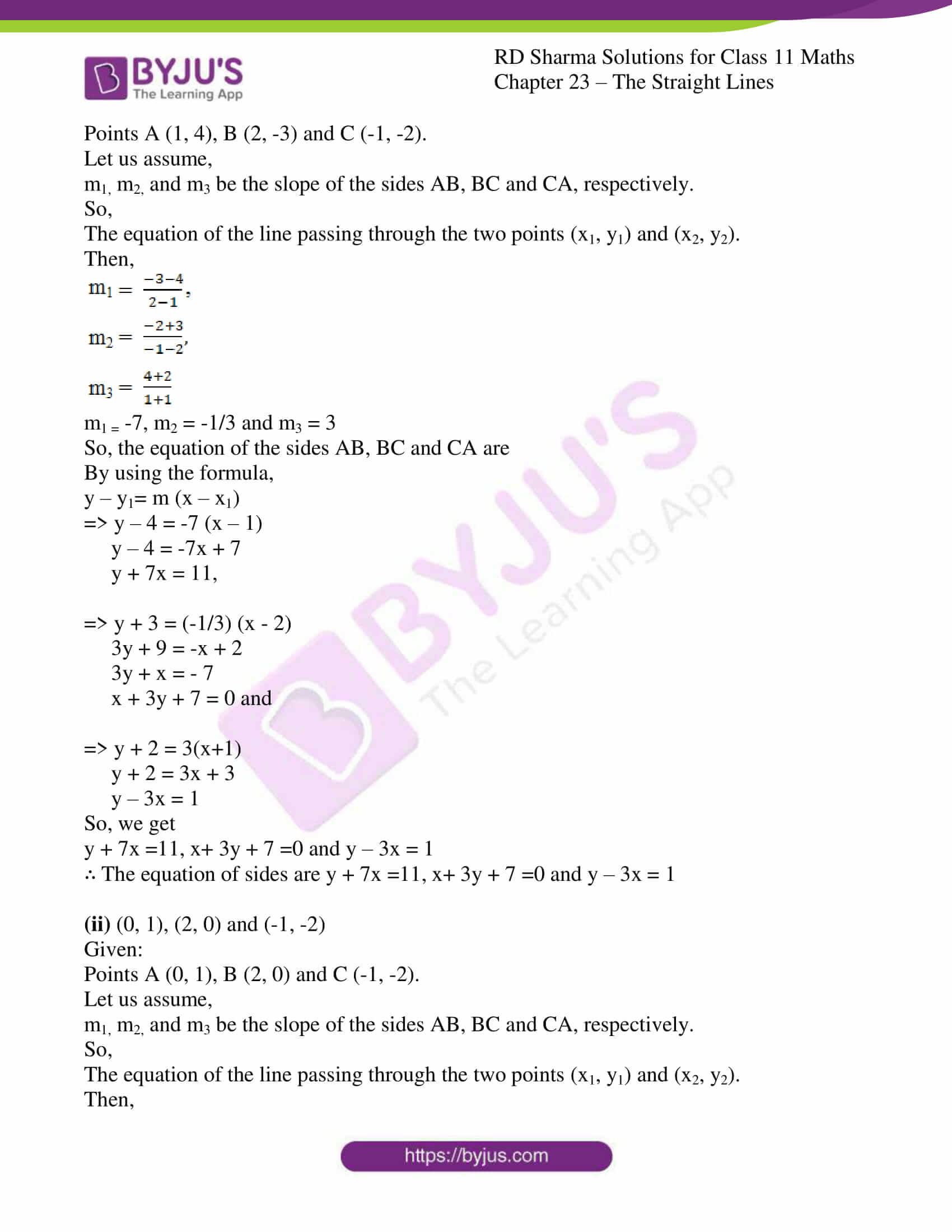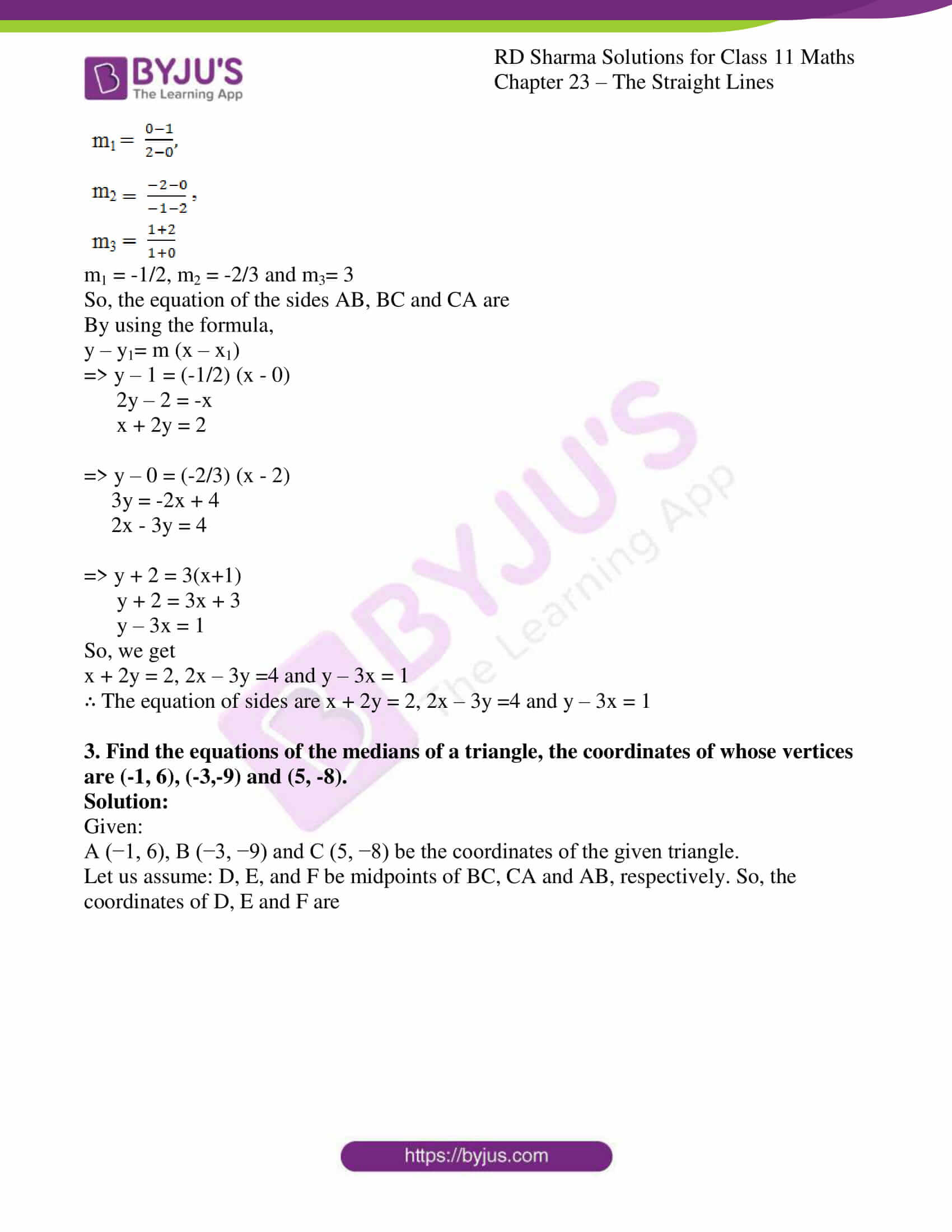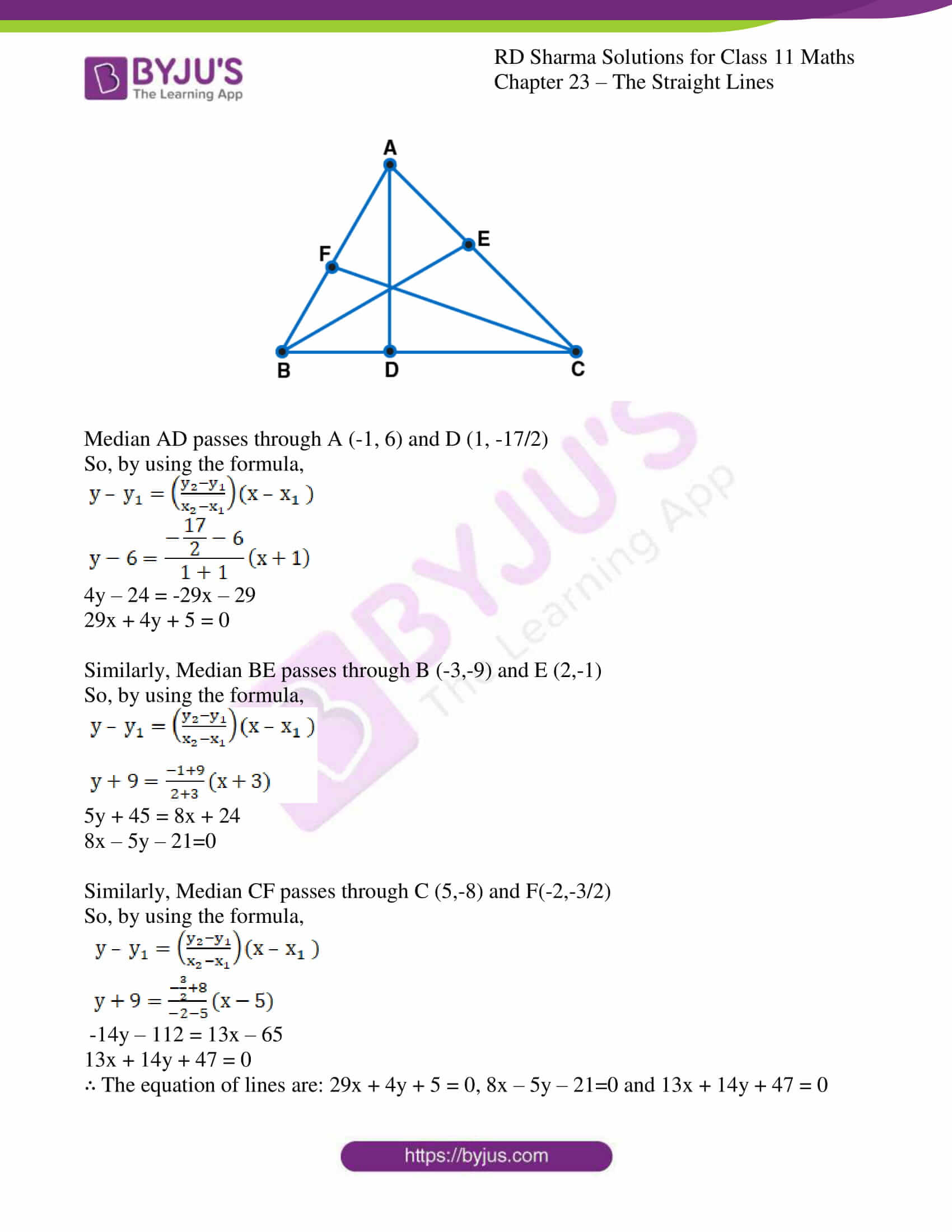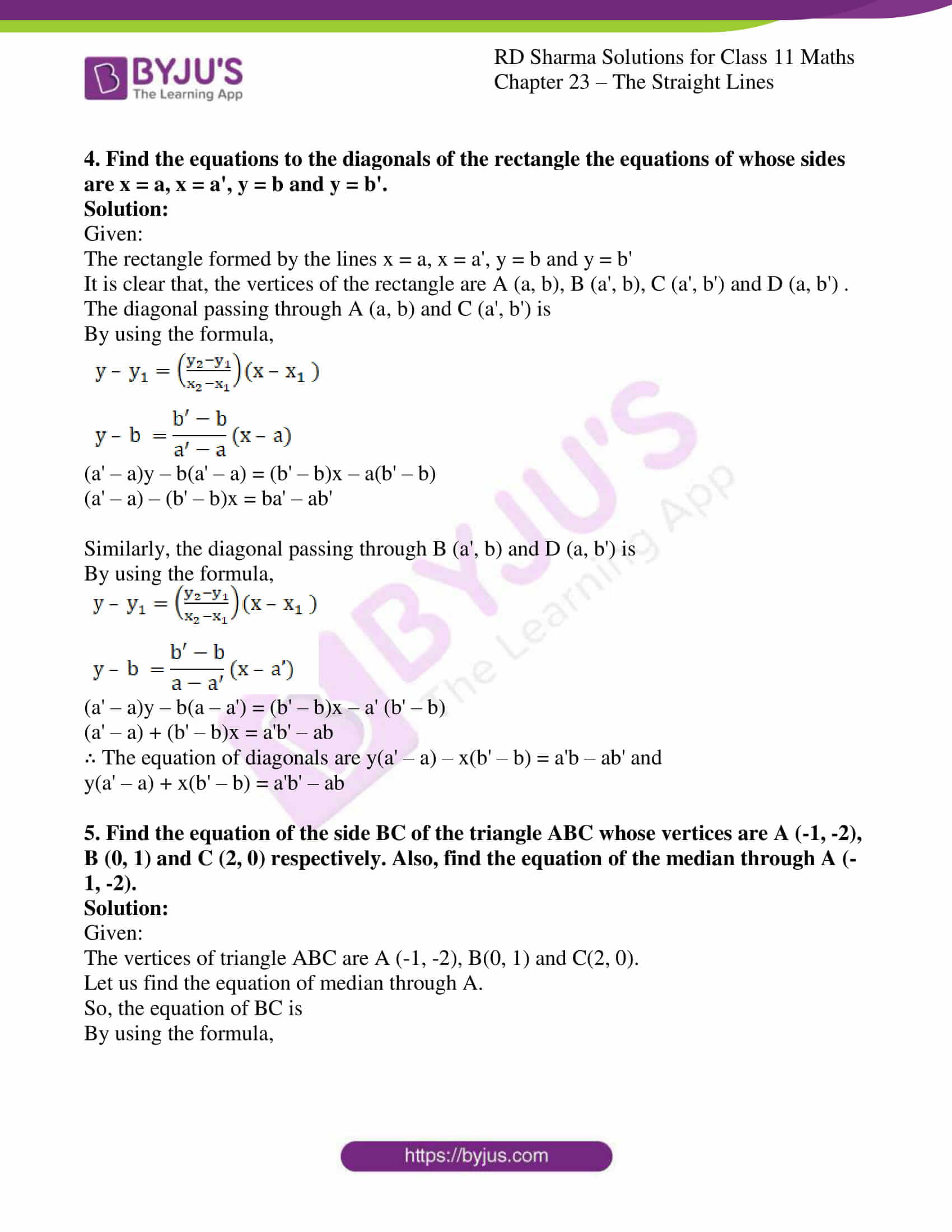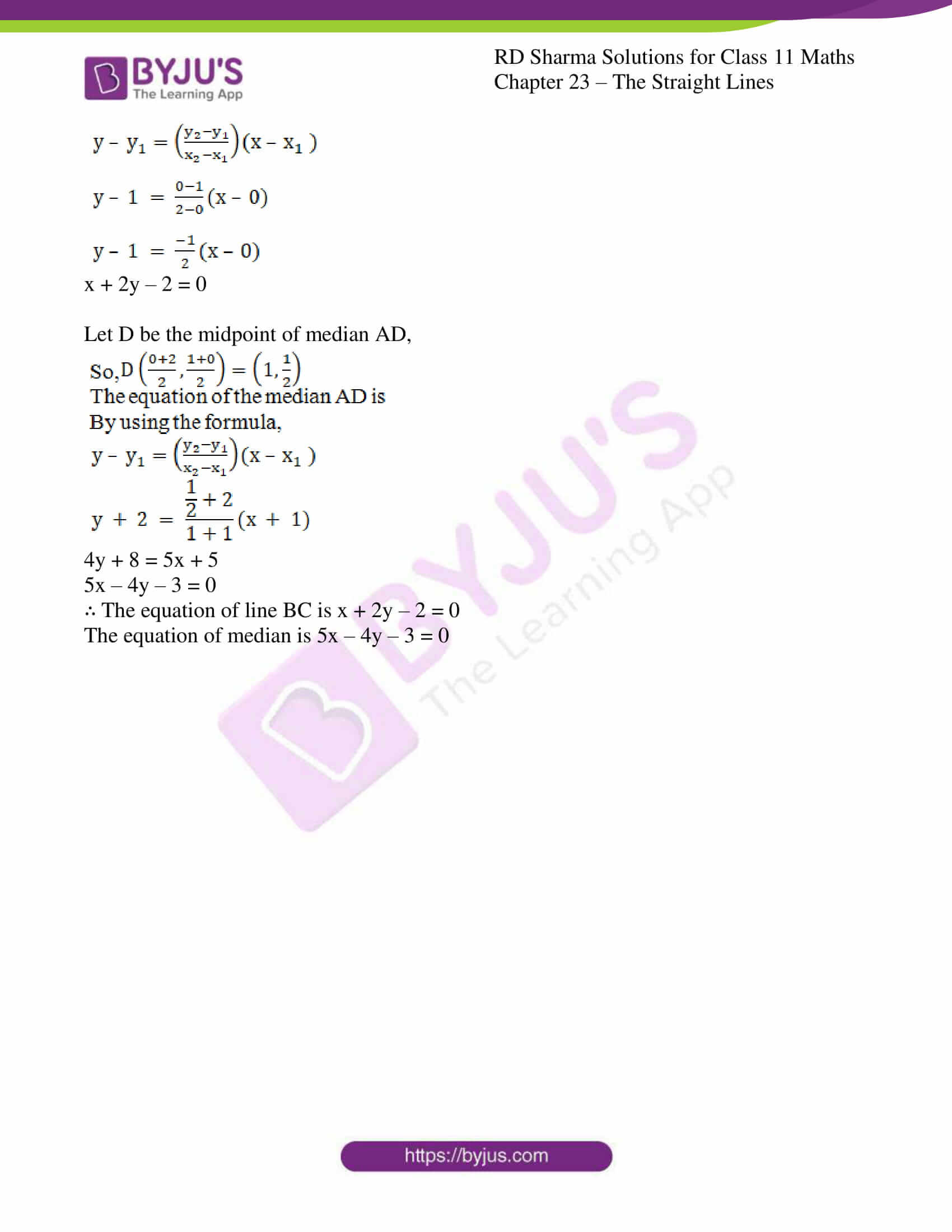### Also, access other exercises of RD Sharma Solutions for Class 11 Maths Chapter 23 – The Straight Lines

Exercise 23.1 Solutions

Exercise 23.2 Solutions

Exercise 23.3 Solutions

Exercise 23.4 Solutions

Exercise 23.6 Solutions

Exercise 23.7 Solutions

Exercise 23.8 Solutions

Exercise 23.9 Solutions

Exercise 23.10 Solutions

Exercise 23.11 Solutions

Exercise 23.12 Solutions

Exercise 23.13 Solutions

Exercise 23.14 Solutions

Exercise 23.15 Solutions

Exercise 23.16 Solutions

Exercise 23.17 Solutions

Exercise 23.18 Solutions

Exercise 23.19 Solutions

### Access answers to RD Sharma Solutions for Class 11 Maths Exercise 23.5 Chapter 23 – The Straight Lines

#### EXERCISE 23.5 PAGE NO: 23.35

1. Find the equation of the straight lines passing through the following pair of points:
(i) (0, 0) and (2, -2)

(ii) (a, b) and (a + c sin α, b + c cos α)

Solution:

(i) (0, 0) and (2, -2)

Given:

(x1, y1) = (0, 0), (x2, y2) = (2, -2)

The equation of the line passing through the two points (0, 0) and (2, −2) is

By using the formula,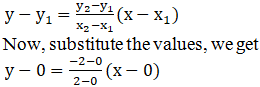y = – x

∴ The equation of line is y = -x

(ii) (a, b) and (a + c sin α, b + c cos α)

Given:

(x1, y1) = (a, b), (x2, y2) = (a + c sin α, b + c cos α)

So, the equation of the line passing through the two points (0, 0) and (2, −2) is

By using the formula,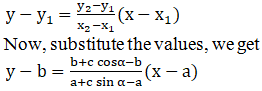y – b = cot α (x – a)

∴ The equation of line is y – b = cot α (x – a)

2. Find the equations to the sides of the triangles the coordinates of whose angular points are respectively:
(i) (1, 4), (2, -3) and (-1, -2)

(ii) (0, 1), (2, 0) and (-1, -2)

Solution:

(i) (1, 4), (2, -3) and (-1, -2)

Given:

Points A (1, 4), B (2, -3) and C (-1, -2).

Let us assume,

m1, m2, and m3 be the slope of the sides AB, BC and CA, respectively.

So,

The equation of the line passing through the two points (x1, y1) and (x2, y2).

Then,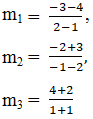m1 = -7, m2 = -1/3 and m3 = 3

So, the equation of the sides AB, BC and CA are

By using the formula,

y – y1= m (x – x1)

=> y – 4 = -7 (x – 1)

y – 4 = -7x + 7

y + 7x = 11,

=> y + 3 = (-1/3) (x – 2)

3y + 9 = -x + 2

3y + x = – 7

x + 3y + 7 = 0 and

=> y + 2 = 3(x+1)

y + 2 = 3x + 3

y – 3x = 1

So, we get

y + 7x =11, x+ 3y + 7 =0 and y – 3x = 1

∴ The equation of sides are y + 7x =11, x+ 3y + 7 =0 and y – 3x = 1

(ii) (0, 1), (2, 0) and (-1, -2)

Given:

Points A (0, 1), B (2, 0) and C (-1, -2).

Let us assume,

m1, m2, and m3 be the slope of the sides AB, BC and CA, respectively.

So,

The equation of the line passing through the two points (x1, y1) and (x2, y2).

Then,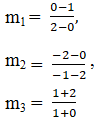m1 = -1/2, m2 = -2/3 and m3= 3

So, the equation of the sides AB, BC and CA are

By using the formula,

y – y1= m (x – x1)

=> y – 1 = (-1/2) (x – 0)

2y – 2 = -x

x + 2y = 2

=> y – 0 = (-2/3) (x – 2)

3y = -2x + 4

2x – 3y = 4

=> y + 2 = 3(x+1)

y + 2 = 3x + 3

y – 3x = 1

So, we get

x + 2y = 2, 2x – 3y =4 and y – 3x = 1

∴ The equation of sides are x + 2y = 2, 2x – 3y =4 and y – 3x = 1

3. Find the equations of the medians of a triangle, the coordinates of whose vertices are (-1, 6), (-3,-9) and (5, -8).

Solution:

Given:

A (−1, 6), B (−3, −9) and C (5, −8) be the coordinates of the given triangle.

Let us assume: D, E, and F be midpoints of BC, CA and AB, respectively. So, the coordinates of D, E and F are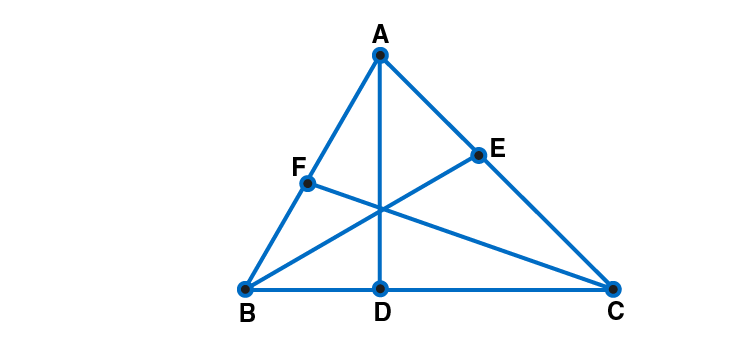Median AD passes through A (-1, 6) and D (1, -17/2)

So, by using the formula,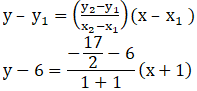4y – 24 = -29x – 29

29x + 4y + 5 = 0

Similarly, Median BE passes through B (-3,-9) and E (2,-1)

So, by using the formula,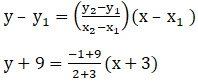5y + 45 = 8x + 24

8x – 5y – 21=0

Similarly, Median CF passes through C (5,-8) and F(-2,-3/2)

So, by using the formula,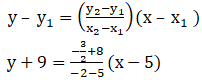-14y – 112 = 13x – 65

13x + 14y + 47 = 0

∴ The equation of lines are: 29x + 4y + 5 = 0, 8x – 5y – 21=0 and 13x + 14y + 47 = 0

4. Find the equations to the diagonals of the rectangle the equations of whose sides are x = a, x = a’, y = b and y = b’.

Solution:

Given:

The rectangle formed by the lines x = a, x = a’, y = b and y = b’

It is clear that, the vertices of the rectangle are A (a, b), B (a’, b), C (a’, b’) and D (a, b’) .

The diagonal passing through A (a, b) and C (a’, b’) is

By using the formula,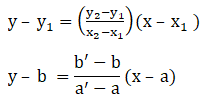(a’ – a)y – b(a’ – a) = (b’ – b)x – a(b’ – b)

(a’ – a) – (b’ – b)x = ba’ – ab’

Similarly, the diagonal passing through B (a’, b) and D (a, b’) is

By using the formula,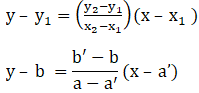(a’ – a)y – b(a – a’) = (b’ – b)x – a’ (b’ – b)

(a’ – a) + (b’ – b)x = a’b’ – ab

∴ The equation of diagonals are y(a’ – a) – x(b’ – b) = a’b – ab’ and

y(a’ – a) + x(b’ – b) = a’b’ – ab

5. Find the equation of the side BC of the triangle ABC whose vertices are A (-1, -2), B (0, 1) and C (2, 0) respectively. Also, find the equation of the median through A (-1, -2).

Solution:

Given:

The vertices of triangle ABC are A (-1, -2), B(0, 1) and C(2, 0).

Let us find the equation of median through A.

So, the equation of BC is

By using the formula,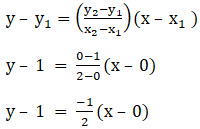x + 2y – 2 = 0

Let D be the midpoint of median AD,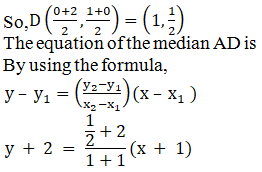4y + 8 = 5x + 5

5x – 4y – 3 = 0

∴ The equation of line BC is x + 2y – 2 = 0

The equation of median is 5x – 4y – 3 = 0